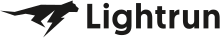• Dev Observability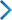• Product• Pricing
• Docs
• Resources• Blog
• Company• Debug Wordle# Stuck on an issue?

Lightrun Answers was designed to reduce the constant googling that comes with debugging 3rd party libraries. It collects links to all the places you might be looking at while hunting down a tough bug.

And, if you’re still stuck at the end, we’re happy to hop on a call to see how we can help out.

## Inconsistent assumptions about vector space (dimension)

See original GitHub issue

### Issue Description

The vector operations on `compas.geometry.basic` (source) are inconsistent in the way they assume the vector space. Some of them work on arbitrary length vectors, while others are hard-coded to 3D vectors, and documentation only refers to support of 3D vectors, even in the cases where the function support arbitrary length.

For example, `dot_vectors`, `add_vectors`, `subtract_vectors`, `scale_vector` and many others work on any length, but `cross_vectors`, `length_vector`, `normalize_vector` and others are hard-coded to 3D.

### Issue Analytics

• State: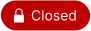• Created 5 years ago

####Top GitHub Comments

1reaction
brgcodecommented, Apr 18, 2018

was hoping you would say that. alright, will do!

0reactions
brgcodecommented, Aug 20, 2018

perhaps we should just remove

• `norm_vector(s)`
• `power_vector(s)`

as these are functions that make more sense in a general vector calculus context and not so much in a geometrical one.

####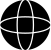Top Results From Across the Web

If \$Ax=b\$ is inconsistent for some \$b\$, what does this tell us ...
Dimension of solution set for Ax=b. But the solution set isn't a vector space so why talk about it's dimensions?
On a Particular Case of the Inconsistent Linear Matrix ... - CORE
We consider the linear matrix equation AX + YB = C where A, B, and C are given matrices of dimensions (r +...
Inconsistency evaluation in pairwise ... - Springer Link
This paper studies the properties of an inconsistency index of a pairwise comparison matrix under the assumption that the index is defined ...
Methods for Finding Bases - TAMU Math
Since the dimension of a space is the number of vectors in a basis, the rank of a matrix is just the number...
Preprocessing Inconsistent Linear System for a Meaningful ...
Abstract Mathematical models of many physical/statistical problems are systems of linear equations~ Due to measurement and possible human.

####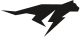Troubleshoot Live Code

Lightrun enables developers to add logs, metrics and snapshots to live code - no restarts or redeploys required.
Start Free

####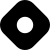Top Related Hashnode Post